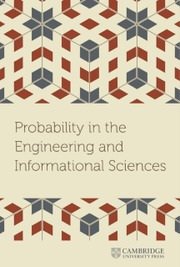Home
Hostname: page-component-544b6db54f-rlmms Total loading time: 0.195 Render date: 2021-10-24T17:04:50.539Z Has data issue: true Feature Flags: { "shouldUseShareProductTool": true, "shouldUseHypothesis": true, "isUnsiloEnabled": true, "metricsAbstractViews": false, "figures": true, "newCiteModal": false, "newCitedByModal": true, "newEcommerce": true, "newUsageEvents": true }Probability in the Engineering and Informational Sciences

# Concentrated matrix exponential distributions with real eigenvalues

Published online by Cambridge University Press:  26 August 2021

## Abstract

Concentrated random variables are frequently used in representing deterministic delays in stochastic models. The squared coefficient of variation ($\mathrm {SCV}$) of the most concentrated phase-type distribution of order$N$ is$1/N$. To further reduce the$\mathrm {SCV}$, concentrated matrix exponential (CME) distributions with complex eigenvalues were investigated recently. It was obtained that the$\mathrm {SCV}$ of an order$N$ CME distribution can be less than$n^{-2.1}$ for odd$N=2n+1$ orders, and the matrix exponential distribution, which exhibits such a low$\mathrm {SCV}$ has complex eigenvalues. In this paper, we consider CME distributions with real eigenvalues (CME-R). We present efficient numerical methods for identifying a CME-R distribution with smallest SCV for a given order$n$. Our investigations show that the$\mathrm {SCV}$ of the most concentrated CME-R of order$N=2n+1$ is less than$n^{-1.85}$. We also discuss how CME-R can be used for numerical inverse Laplace transformation, which is beneficial when the Laplace transform function is impossible to evaluate at complex points.

Type
Research Article
Information

## Access options

Get access to the full version of this content by using one of the access options below. (Log in options will check for institutional or personal access. Content may require purchase if you do not have access.)

## References

Abate, J. & Whitt, W. (2006). A unified framework for numerically inverting Laplace transforms. INFORMS Journal on Computing 18(4): 408421.CrossRefGoogle Scholar
Abate, J., Choudhury, G.L., & Whitt, W. (2000). An introduction to numerical transform inversion and its application to probability models. In W. K. Grassmann (ed.), Computational probability. Boston, MA: Springer US, pp. 257–323.CrossRefGoogle Scholar
Airapetyan, R.G. & Ramm, A.G. (2000). Numerical inversion of the Laplace transform from the real axis. Journal of Mathematical Analysis and Applications 248(2): 572587.CrossRefGoogle Scholar
Aldous, D. & Shepp, L. (1987). The least variable phase type distribution is Erlang. Stochastic Models 3: 467473.Google Scholar
Éltető, T., Rácz, S., & Telek, M. (2006). Minimal coefficient of variation of matrix exponential distributions. In 2nd Madrid Conference on Queueing Theory, Madrid, Spain, July 2006.Google Scholar
Gaver, D.P. (1966). Observing stochastic processes and approximate transform inversion. Operations Research 14: 444459.CrossRefGoogle Scholar
Hansen, N. (2006). The CMA evolution strategy: a comparing review. In J. A. Lozano, P. Larrañaga, I. Inza, & E. Bengoetxea (eds), Towards a new evolutionary computation. Springer, pp. 75–102.CrossRefGoogle Scholar
Hansen, N. (2009). Benchmarking a BI-population CMA-ES on the BBOB-2009 function testbed. In Proceedings of the 11th Annual Conference Companion on Genetic and Evolutionary Computation Conference: Late Breaking Papers. ACM, pp. 2389–2396. https://doi.org/10.1145/1570256.1570333CrossRefGoogle Scholar
Horváth, I., Horváth, G., Al-Deen Almousa, S., & Telek, M. (2019). Numerical inverse Laplace transformation by concentrated matrix exponential distributions. Performance Evaluation 137: 102067.CrossRefGoogle Scholar
Horváth, G., Horváth, I., & Telek, M. (2020). High order concentrated matrix-exponential distributions. Stochastic Models 36(2): 176192.CrossRefGoogle Scholar
Keller, H.B. & Pereyra, V. (1978). Symbolic generation of finite difference formulas. Mathematics of Computation 32(144): 955971.CrossRefGoogle Scholar
Koskela, H., Kilpeläinen, I., & Heikkinen, S. (2004). Evaluation of protein$^{1}5$N relaxation times by inverse Laplace transformation. Magnetic Resonance in Chemistry 42(1): 6165.CrossRefGoogle Scholar
Nishiyama, Y., Frey, M.H., Mukasa, S., & Utsumi, H. (2010).$^{1}3$C solid-state NMR chromatography by magic angle spinning$^{1}$H$T_1$ relaxation ordered spectroscopy. Journal of Magnetic Resonance 202(2): 135139.CrossRefGoogle Scholar
Stehfest, H. (1970). Algorithm 368: Numerical inversion of Laplace transforms [D5]. Communications of the ACM 13(1): 4749.CrossRefGoogle Scholar
Valko, P. & Abate, J. (2004). Comparison of sequence accelerators for the Gaver method of numerical Laplace transform inversion. Computers and Mathematics with Applications 48: 629636.CrossRefGoogle Scholar

# Send article to Kindle

Note you can select to send to either the @free.kindle.com or @kindle.com variations. ‘@free.kindle.com’ emails are free but can only be sent to your device when it is connected to wi-fi. ‘@kindle.com’ emails can be delivered even when you are not connected to wi-fi, but note that service fees apply.

Find out more about the Kindle Personal Document Service.

Concentrated matrix exponential distributions with real eigenvalues
Available formats
×

# Send article to Dropbox

To send this article to your Dropbox account, please select one or more formats and confirm that you agree to abide by our usage policies. If this is the first time you use this feature, you will be asked to authorise Cambridge Core to connect with your <service> account. Find out more about sending content to Dropbox.

Concentrated matrix exponential distributions with real eigenvalues
Available formats
×

# Send article to Google Drive

To send this article to your Google Drive account, please select one or more formats and confirm that you agree to abide by our usage policies. If this is the first time you use this feature, you will be asked to authorise Cambridge Core to connect with your <service> account. Find out more about sending content to Google Drive.

Concentrated matrix exponential distributions with real eigenvalues
Available formats
×
×